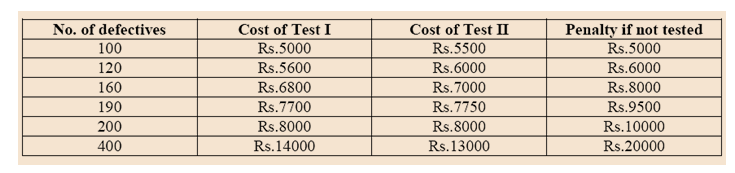Directions: Prakash has to decide whether or not to test a batch of 1000 widgets before sending them to the buyer. In case he decides to test, he has two options:
(a) Use test I
(b) Use test II.

Test I cost Rs. 2 per widget. However, the test is not perfect. It sends 20% of the bad ones to the buyer as good.
Test II costs Rs. 3 per widget. It brings out all the bad ones. A defective widget identified before sending can be corrected at a cost of Rs. 25 per widget.
All defective widgets are identified at the buyer’s end and penalty of Rs. 50 per defective widget has to be paid by Prakash.

Question 1. Prakash should not test if the number of bad widgets in the lot is:
(a) less than 100
(b) more than 200
(c) between 120 & 190
(d) Cannot be found out.

Question 2. If there are 120 defective widgets in the lot, Prakash:
(a) should either use Test I or not test.
(b) should either use Test II or not test.
(c) should use Test I or Test II.
(d) should use Test I only.

Question 3. If the number of defective widgets in the lot is between 200 and 400, Prakash:
(a) may use Test I or Test II
(b) should use Test I only.
(c) should use Test II only
(d) cannot decide.

Question 4. If Prakash is told that the lot has 160 defective widgets, he should:
(a) use Test I only
(b) use Test II only.
(c) do no testing.
(d) either use Test I or do not test.

Question 5. If there are 200 defective widgets in the lot, Prakash:
(a) may use either Test I or Test II
(b) should use Test I or not use any test
(c) should use Test II or not use any test.
(d) cannot decide.

Answer 1. (a) Let the total number of bad widgets be= A

The total number of good widgets= 1000 –A

If he takes test I, his total cost will be = Rs. 2(1000) + 25 X 0.8A + 50 X 0.2A

If he takes test II, his total cost will be : Rs. 3(1000) + 25 X A

According to the conditions given, testing will be worth is the cost of testing is less than the cost of penalty levied on the defective pieces.

In order to figure out the answers, let us chart out all the options given in the answer options and build a table for the given values:From the above table, it is clear that if the number of defectives more than 100, the cost of testing is cheaper than the penalty. For 100 defectives, the cost of penalty is the same as that for testing. It is obvious that for number of defectives above 100 cost of any testing is cheaper than the penalty. Thus, below 100 defectives, the penalty cost is will less than the cost of testing and hence, it is not worth testing.

Answer 2. (d) We can use the table above to figure out the answer. For 120 widgets, he should go for test I as it is cheaper.

Answer 3. (c) We can use the table above to figure out the answer. From the table, we can find that if the number of defectives is between 200 & 400, he should go for Test II as it is cheaper.

Answer 4. (a) Again, we use the table above. If there are 160 defectives, he should use test I as it is cheaper.

Answer 5. (a) Using the table above, we can see that if there are 200 defective widgets in the lot, the cost for Test I or II is the same (Rs. 8000). Thus, Prakash may use either Test I or Test II.